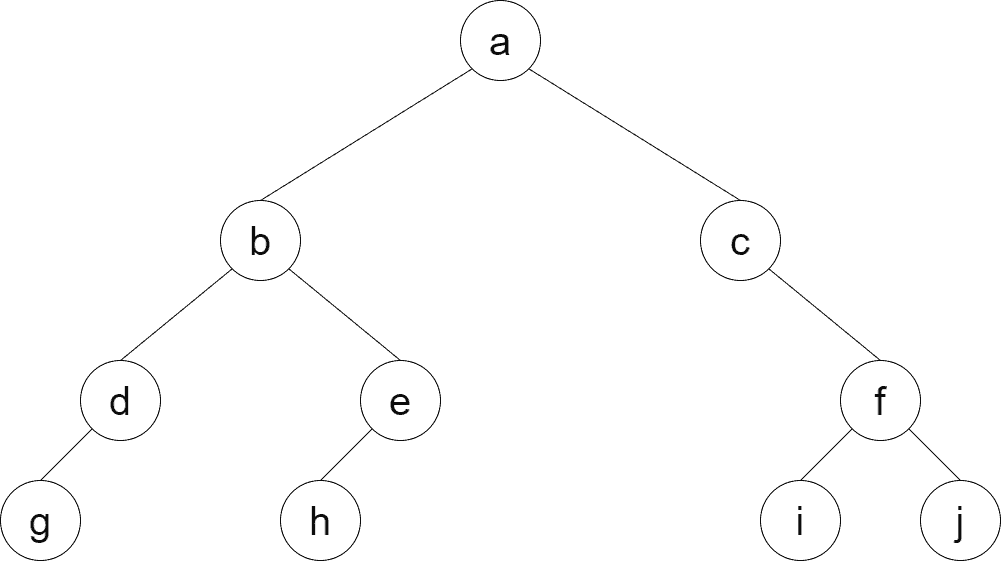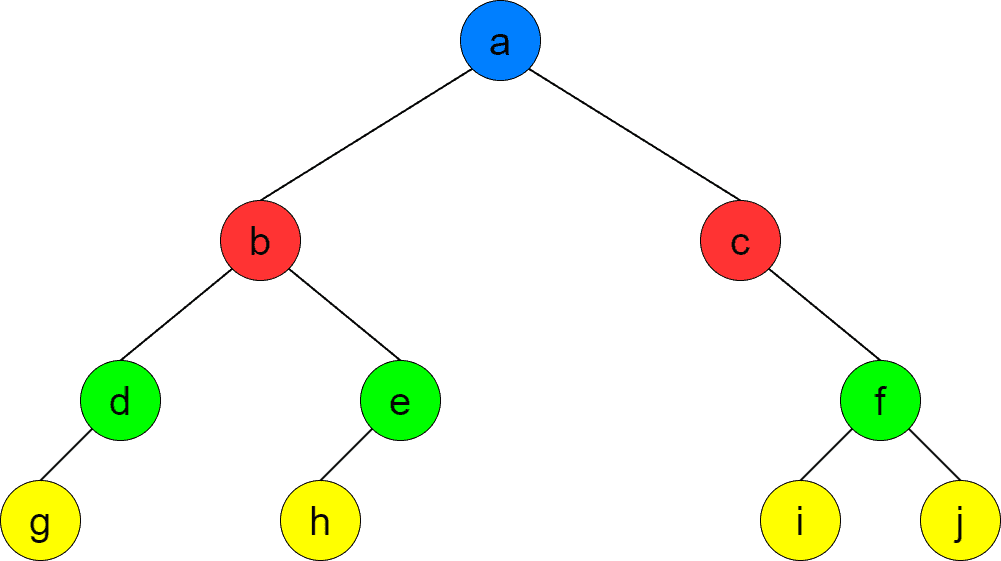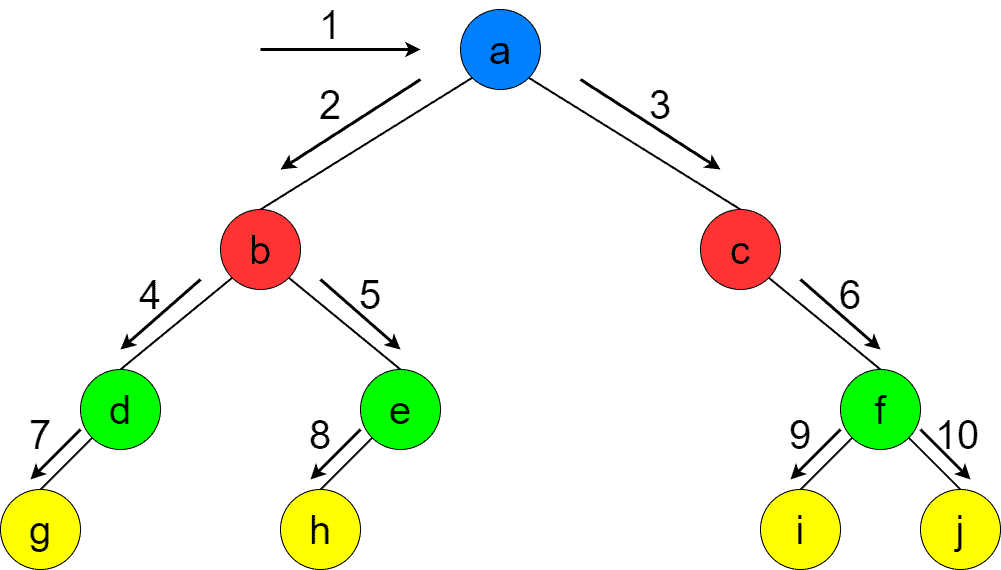## 1. Introduction

In this tutorial, we’ll discuss the level-order traversal of a binary tree. It’s a way to iterate over the nodes of a binary tree, similar to more traditional in-order, pre-order, and post-order traversals.

## 2. Problem Statement

Assume we have a binary tree. We want to visit its nodes by levels, i.e., first visit the root node, then the nodes at the second level, then at the third level, etc.

Let’s consider the binary tree below:It has four levels, shown below in different colors:Level-order traversal helps us visit nodes by levels. It first visits the root node, its children, its grandchildren, and so on. The picture below shows the order of the node traversal:Generally, we don’t care much about the order of the nodes in the same level, i.e., nodecould have been visited before. Nevertheless, we usually traverse a level from left to right. So, when running the level-order traversal of the sample tree above, we’ll get the alphabetical sequence->->->->->->->->->.

## 3. Algorithm Description

Pre-order, in-order and post-order traversals are DFS-based algorithms. In contrast to them, level-order traversal is based on BFS.

Level-order traversal maintains a pool of current nodes. Initially, the pool contains only the root node. At each step, we iterate over the nodes in the pool and collect their children. The pool’s content is dismissed, and the gathered children are added. The process continues until there’re nodes in the tree. It’s not hard to convince ourselves that the process guarantees the nodes are visited level by level.

We can implement level-order traversal both iteratively and recursively.

### 3.1. Iterative Level-order Traversal

As we’ve mentioned, level-order traversal is BFS-based. That effectively means we’re going to use a queue to maintain the current pool of nodes. The front of the queue consists of the nodes to be processed. As we pop the nodes, we push their children into the queue. When the current level nodes are all popped out, the next level nodes are automatically moved to the front of the queue.

Let’s demonstrate the idea with the help of the pseudocode:Actually, the pseudocode above is literally a BFS traversal of a binary tree starting from the root node. Assuming there’renodes in the tree, the time complexity isas we simply visit each node once. The space complexity isas well because the queue may contain approximately half of the nodes at some point during the algorithm. It becomes clear if we imagine a complete binary tree. The last level containsnodes, whereis the tree’s height., so the last level contains more nodes than the rest of the tree (assuming the last level is complete).

### 3.2. Recursive Level-order Traversal

The recursive version’s idea is to process the current nodes, collect their children and then continue the recursion with the collected children. The iterative version uses a queue to maintain the current nodes, while the recursive version may use any structure to persist the nodes. We’ll use a single linked list for our purpose.

We can find the pseudocode for the recursive level-order traversal below:Each recursive invocation processes the nodes of the current level collects the next-level nodes, and invokes itself for those nodes. The time and space complexity is the same as for the iterative version.

## 4. Applications

In this chapter, we discuss some of the applications of level-order traversal.

### 4.1. Finding the Level of a Node

Having a node, we want to find the level (or the height) at which the node resides. For simplicity, we compare the nodes to see if they’re equal. Other applications may define node equality using node IDs or values in nodes, but that fact doesn’t change the algorithm.

Let’s augment the recursive version of level-order traversal to solve the problem:The invocation of the function takes the form:. As we can see, the pseudocode above slightly modifies recursive level-order traversal.

### 4.2. Counting the Number of Nodes at a Level

Another interesting problem is counting the number of nodes at a certain level. Again, we can solve the problem by slightly modifying recursive level-order traversal. Let’s jump to the pseudocode:Similarly to the first algorithm, we invoke the method with the root node:.

Obviously, the time and space complexity of both algorithms is, whereis the number of nodes in the tree.

## 5. Conclusion

In this tutorial, we’ve discussed the level-order traversal of binary trees. It’s a BFS-based algorithm that visits the tree nodes level by level. We’ve also provided the pseudocode for both iterative and recursive versions of level-order traversal. Finally, we’ve reviewed some practical applications of the traversal algorithm.